# Statistical Graphics for Multivariate Data

Michael Friendly, York University

Paper presented at the SAS SUGI 16 Conference, Apr, 1991

# Abstract

This paper presents an overview of graphical methods for displaying multivariate data. These methods are described in detail in my book, The SAS® System for Statistical Graphics. In particular, I illustrate the design and implementation of custom graphic displays for:
• adding more variables to a scatterplot (glyph plots)
• plotting all pairs of variables (scatterplot matrix)
• detecting clusters (star plots)
• plotting observations and variables together (biplot)
• assessing multivariate normality
• detecting outliers
Most of these methods are implemented as general SAS macros, which are included in the book.

# Introduction

Graphs are inherently two-dimensional. Some ingenuity is therefore required to display the relationships of three or more variables on a flat piece of paper. All multivariate graphics require changing or expanding the familiar visual metaphors we use for two variables, and a wide variety of methods have been developed. It is often useful to apply several of these to a given set of data.

While many important new graphical techniques for multivariate data have recently been developed (e.g., Barnett, 1981; Chambers et al., 1983) there is usually a long lag before they are implemented in a widely accessible form. SAS System for Statistical Graphics, First Edition designed to fill this gap. The primary goals of the book are to survey the kinds of graphic displays that are most useful for different questions and data, and to show how can these displays be done with the SAS System. Many of the graphical methods described are implemented as general SAS macro programs which can be used with any set of data. See Friendly (1990) for an overview of the book.

This paper describes the design and implementation of some informal, exploratory techniques for displaying three or more variables in one plot. These methods are illustrated with data on the price, weight, gas mileage and other measures of size and performance on 74 makes of automobiles (Chambers et al., 1983).

# Glyph plots

The simplest extension of the ordinary scatterplot involves choosing two primary variables for a scatterplot, and representing additional variables in a glyph symbol used to plot each observation. The additional variables can be represented by properties such as size, color, shape, length and direction of lines.

The Annotate facility provides a relatively easy way to design your own glyph symbols. The example described in this section, inspired by Nicholson & Littlefield (1983), uses a ray glyph --a whisker line whose length, angle, and color vary--to display two quantitative variables and one categorical variable in addition to the two front variables of an ( x , y ) plot.

The plot, shown in Figure 1, displays the relationship between WEIGHT and PRICE of automobiles in the foreground variables. As indicated in the inset variable key, the length of the whisker line from each point is proportional to gas mileage, MPG, while the angle from the horizontal is proportional to ratings of REPAIR record. The region of origin of each automobile model is coded by both the shape of the marker and the color of the symbol. (The effect of color unfortunately is lost in a monochrome display.)

Figure 1 shows gas mileage decreases (shorter rays) as WEIGHT and PRICE increase; low weight cars also tend to have better REPAIR records (larger ray angle).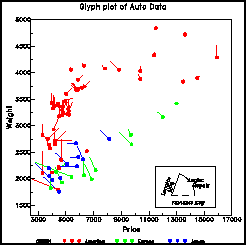Figure 1: Glyph plot of Auto data

The glyph symbol is constructed in the following steps:

1. The REPAIR and MPG variables are first scaled to (0, 1) using the minimum and maximum values from a PROC MEANS output data set. The scaled repair record variable, P2, determines the ANGLE, which is allowed to go from 0 to 180°. The maximum ray length was set at 500 data units, a value determined by trial and error.
2. The foreground variables, WEIGHT and PRICE determine the Annotate variables X and Y for a MOVE operation, and the conversion of ray length (500 * P1) and angle from polar to rectangular coordinates determines X and Y for the DRAW operation.
```proc means data=auto min max ;
var mpg repair;
output out=range min=mpgmin repmin
max=mpgmax repmax;
data glyph;
set auto;
length color function \$8 ;
if _n_=1 then set range;
xsys='2'; ysys='2';

/* Scale glyph variables to (0,1) */
p1 = (mpg - mpgmin) / (mpgmax - mpgmin);
p2 = (repair - repmin) / (repmax - repmin);

x = price; y = weight;  x_to_y = 4;
function = 'MOVE'; output;
angle = 180 * p2 * arcos(-1)/180;
x = x + 700* p1 * cos(angle) * x_to_y;
y = y + 700* p1 * sin(angle);
select;
when (origin ='A')  color = 'RED';
when (origin ='E')  color = 'GREEN';
when (origin ='J')  color = 'BLUE';
end;
function = 'DRAW'; output;
```

# Scatterplot Matrix

Glyph plots are useful for 3-5 variables, but they do not generalize easily to an arbitrary number of variables. Figure 2 is an example of a scatterplot matrix , a technique which can be use for any number of variables. It shows the relations among the variables PRICE, WEIGHT, MPG, and REPAIR in the AUTO data, with the region of origin determining the plotting symbol. In this plot we can see:
• moderately strong (negative) correlations between MPG and both PRICE and WEIGHT.
• High mileage cars also tend to have better REPAIR records and are mostly Japanese.
• A positive relation between PRICE and WEIGHT for all three regions of origin, with US models generally heavier.
• The relationship between PRICE and REPAIR record is complex, and possibly nonlinear.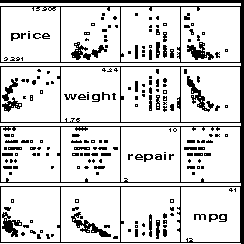Figure 2: Scatterplot matrix for AUTO data. US models: circles, European models: squares, Japanese models: stars.

## SCATMAT macro

The scatterplot matrix is implemented as a SAS macro, SCATMAT, which constructs the plot for any number of variables.

For p variables, x sub 1 , ... , x sub p, the scatterplot matrix is a p x p array in which the cell in row i, column j contains the plot of x sub i against x sub j. The diagonal cells are used for the variable names and scale markings. In the SAS macro language, this can be done with two nested %DO loops. which call PROC GPLOT for each pair of variables. The set of p times p plots is then displayed with a PROC GREPLAY step, which is also constructed by the SCATMAT macro.

The SCATMAT macro also allows a class or grouping variable in the data set to be used to determine the shape and color of the plotting symbol. The parameters and default values for the SCATMAT macro are shown below.

```%macro SCATMAT(
data =_LAST_,       /* data set plotted  */
var  =_NUMERIC_,    /* variables plotted */
group=,             /* grouping variable */
symbols=%str(- + : \$ = X _ Y),
colors=BLACK RED GREEN BLUE BROWN
YELLOW ORANGE PURPLE,
gout=GSEG);
```

The plot in Figure 2 is produced using the SCATMAT macro as shown below. Region of origin is used to define the plotting symbol.

```%scatmat(data=auto,
var=price weight repair mpg,
symbols=+ SQUARE STAR,
colors=RED GREEN BLUE,
group=origin );
```

# Star plots

Star plots (Chambers, et al., 1983, pp. 158-162) are a useful way to display multivariate observations with an arbitrary number of variables. Each observation is represented as a star-shaped figure with one ray for each variable. For a given observation, the length of each ray is made proportional to the size of that variable. Star plots differ from glyph plots in that all variables are used to construct the plotted star figure; there is no separation into foreground and background variables. Instead, the star-shaped figures are usually arranged in a rectangular array on the page. It is somewhat easier to see patterns in the data if the observations are arranged in some non-arbitrary order, and if the variables are assigned to the rays of the star in some meaningful order. Figure 3 shows a star plot of the 12 numeric variables in the automobiles data. These 12 variables are arranged around the perimeter as shown in the variable assignment key in Figure 4.Figure 3: Star plot of automobile data. Each star represents one car model; each ray in the star is proportional to one variable. Only the 8 lightest (top two rows) and 8 heaviest models (bottom two rows) are shown.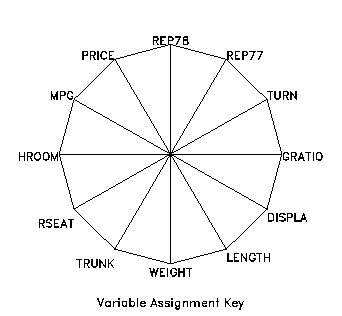Figure 4: Variable assignment key for star plot. The variables at the sides and bottom are related to size; the others relate to price and performance.

The star plot is constructed using the Annotate facility with PROC GSLIDE. To make this procedure general, it has been written as a SAS macro program, STARS, which takes the following parameters:

```%macro STARS(
data=_LAST_,    /* Data set plotted       */
var=_NUMERIC_,  /* Variables, as ordered  */
/* around the star        */
id=,            /* Observation identifier */
minray=.1,      /* Minimum ray length 0-1 */
across=5,       /* stars across a page    */
down=6 );       /* stars down a page      */
```
The star plot is most useful when all of the variables have their scales aligned in the same direction so that increasing values have a similar meaning for all variables. For the AUTO data, this means that large values of a variable should reflect a "better" car and appear as long rays. To do this, the sign of PRICE, TURN, and GRATIO were changed before using STARS by this DATA step:
```proc sort;
by weight;
data autot;
set auto;
price = -price;   /* make large values  */
turn  = -turn;    /* represent 'good' cars*/
gratio= -gratio;
if _n_ <= 8 or _n_ <  66-8 ;
```
Then, STARS was invoked by the following program lines to produce Figure 3 and Figure 4.
```%stars(data=autot,
vars= gratio turn rep77 rep78 price mpg
hroom rseat trunk weight length displa,
id=model,minray=.1);
```
The dominant pattern in Figure 3 is that the star symbols in the top rows have long rays on the top (good price and performance) and short rays on the bottom (small in size variables), but the reverse is generally true for the heaviest models in the bottom rows.

Note that in the star plot we tend to see the configural properties of the collection of variables represented for each observation, and that this perception is affected by the ordering of variables around the perimeter and by the arrangement of stars on the page. Other arrangements might lead to noticing other features of the data, so it might be useful to try several alternatives.

# Biplot

Scatterplot matrices, glyphs, and stars, all focus attention on the observations and portray the relations among the variables only implicitly. The biplot, proposed by Gabriel (1971; 1981), displays the observations and variables in the same plot, in a way that depicts their joint relationships.

The biplot is based on the idea that any data matrix, Y ( n x p ), can be represented approximately in d dimensions (d is usually 2 or 3) as the product of a two matrices, A ( n x d ), and B ( p x d ),

Y approx A B prime . (1)
The rows of A represent the observations in a two- (or three-) dimensional space, and the columns of B prime represent the variables in the same space. The prefix "bi" in the name biplot stems from the fact that both the observations and variables are represented in the same plot, rather than to the fact that a two-dimensional representation is usually used.

The approximation used in the biplot is like that in principal components analysis: the biplot dimensions account for the greatest possible variance of the original data matrix. In the biplot display,

• the observations are usually plotted as points. The configuration of points is essentially the same as scores on the first two principal components.
• the variables are plotted as vectors from the origin. The angles between the vectors represent the correlations among the variables.

## BIPLOT macro

A simple biplot can be constructed from the output data sets of PROC PRINCOMP. A more general version was implemented as a SAS macro in PROC IML to allow different scalings (GH, JK, and symmetric factorizations) of the variable and observation points. The BIPLOT macro takes the following parameters:
```%macro BIPLOT(
data=_LAST_,     /* Data set for biplot     */
var =_NUMERIC_,  /* Variables for biplot    */
id  =ID,         /* Observation ID variable */
dim =2,          /* Number of dimensions    */
factype=SYM,     /* factor type: GH|SYM|JK  */
scale=1,         /* Scale factor for vars   */
out =BIPLOT,     /* Biplot coordinates      */
anno=BIANNO,     /* Annotate labels         */
std=MEAN,        /* Standardize: NO|MEAN|STD*/
pplot=YES);      /* Produce printer plot?   */
```
The number of biplot dimensions is specified by the DIM= parameter and the type of biplot factorization by the FACTYPE= value. The BIPLOT macro constructs two output data sets, identified by the parameters OUT= and ANNO=. The macro will produce a printed plot (if PPLOT=YES), but leaves it to the user to construct a PROC GPLOT plot, since the scaling of the axes should be specified to achieve an appropriate geometry in the plot.

A two-dimensional biplot of the auto data is shown in Figure 5. The horizontal dimension represents the size variables, LENGTH, WEIGHT, and DISPLAcement. The negative relationship of these variables to gas mileage (MPG) and gear ratio is shown by vectors in the opposite direction. The vertical dimension reflects mainly the repair record variable, with moderate contributions from PRICE, trunk size and head room.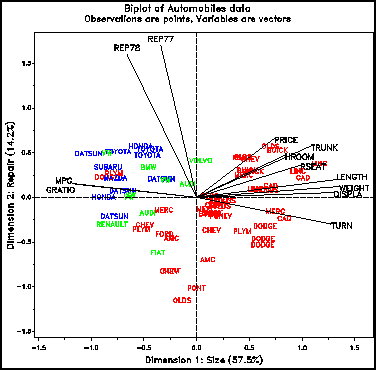Figure 5: Two-dimensional biplot of Auto data

# Assessing multivariate normality

The graphical methods described above make few assumptions about the data. Confirmatory (inferential) methods for multivariate data, however, are almost universally based on the assumption that the data or residuals have a multivariate normal distribution. This section describes a chi² probability plot for determining whether this assumption is reasonable. A robust version of this plot, described in the next section is used to detect multivariate outliers.

The basic principle is to calculate a quantity from each multivariate observation, such that this quantity follows a known probability distribution when the data follows the multivariate normal distribution. Then, a Q-Q plot of observed quantiles against quantiles of the reference distribution will plot as a straight line when the data is multivariate normal (see Gnanadesikan, 1977).

The simplest graphical display for multivariate normality uses the generalized (Mahalanobis) squared distance between the i-th observation vector x sub i prime = ( x sub i1 , x sub i2 , ... , x sub ip ) and the mean vector x bar for the total sample, defined as

D sub i² = ( x sub i - x bar ) prime S sup < - 1 < ( x sub i - x bar ) (2)
where S is the p x p sample variance covariance matrix. With p variables, D sub i² is distributed approximately as chi² with p degrees of freedom for large samples from the multivariate normal distribution. Therefore, a Q-Q plot of the ordered distance values, D sub (i)² against the corresponding quantiles of the chi² ( p ) distribution should yield a straight line through the origin for multivariate normal data.

The chi² quantiles can be calculated in SAS with the GAMINV function or with the CINV function. The D sub i² can be calculated easily by transforming the data to standardized principal components scores. For, if z sub i is the vector of standardized principal component scores corresponding to x sub i, then the squared distance is just the sum of squares of the elements in z sub i,

D sub i² = z sub i prime z sub i = Sigma from j=1 to p z sub ij² . (3)

Thus, the squared distances for variables X1-X5 (say) can be calculated with PROC PRINCOMP and a DATA step as,

```proc princomp std out=pc;
var x1-x5;
data pc;
set pc;
dsq = uss(of prin1-prin5);
```

The chi² probability plot for the auto data is shown in Figure 6. There is some indication of departure from the reference line in the upper right, but it is probably not great enough to reject the assumption of multivariate normality for practical purposes.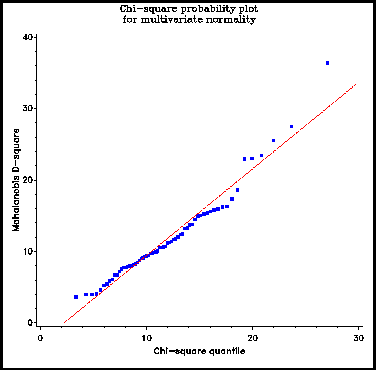Figure 6: Chi-square probability plot for AUTO data

# Detecting multivariate outliers

In the chi² probability plot, potential outliers appear as points in the upper right which are substantially above the line for the expected chi² quantiles. Unfortunately, like all classical (least squares) techniques, the chi² plot for multivariate normality is not resistant to the effects of outliers. A few discrepant observations not only affect the mean vector, but also inflate the variance covariance matrix. Thus, the effect of the few wild observations is spread through all the D ² values.

One solution is to use multivariate trimming (Gnanadesikan, 1977), to calculate squared distances which are not affected by potential outliers. This is an iterative process where, on each iteration, observations with the largest D sup 2 values are temporarily set aside, and the trimmed mean, x bar sub (-) and trimmed variance covariance matrix, S sub (-) are computed from the remaining observations. Then new D ² values are computed using the robust mean and covariance matrix,

D sub i² = ( x sub i - x bar sub (-) ) prime S sub (-) sup < - 1 < ( x sub i - x bar sub (-) ) . (4)
The effect of trimming is that observations with large distances do not contribute to the calculations for the remaining observations. This process is effected using the WEIGHT statement in PROC PRINCOMP, by setting to zero the weight for any observation with a significantly large chi².

## OUTLIER macro

This scheme for outlier detection has been implemented in a general SAS macro, OUTLIER. The arguments to the macro are shown below. PVALUE is the probability, such that an observation is trimmed when its D ² has a probability less than PVALUE. The macro produces an output data set (the OUT= parameter) containing the variables DSQ and EXPECTED (the chi² quantile) in addition to the input variables.
```macro OUTLIER(
data=_LAST_,    /* Data set to analyze       */
var=_NUMERIC_,  /* input variables           */
id=,            /* ID variable for labels    */
out=CHIPLOT,    /* Output dataset for plot   */
pvalue=.1,      /* Prob < pvalue -> weight=0 */
passes=2,       /* Number of passes          */
print=YES);     /* Print OUT= data set?      */

```
The OUTLIER macro is applied to the auto data with the following macro call:
```data AUTO;
%outlier(data=auto,
var=price mpg repair hroom rseat trunk
weight length turn displa gratio,
pvalue=.05,
id=model, out=chiplot);
```
The untrimmed plot ( Figure 6) showed only a slight tendency for points in the upper right to drift away from the reference line. The iterative trimming procedure, however, indicates that on the final pass six observations had probability values more extreme than the .05 cutoff used (see Figure 7). The plot of DSQ vs. EXPECTED values from the OUTLIER macro is shown in Figure 8.
```
PASS    MODEL                  DSQ       PROB

1      AMC PACER            22.7827    0.01896
1      CHEV. CHEVETTE       23.5344    0.01485
1      VW RABBIT DIESEL     25.3503    0.00810
1      VW DASHER            36.3782    0.00015
2      AMC PACER            34.4366    0.00031
2      CHEV. CHEVETTE       36.7623    0.00013
2      PLYM. CHAMP          20.9623    0.03376
2      VW RABBIT DIESEL     44.2961    0.00001
2      VW DASHER            78.5944    0.00000

```

Figure 7: Observations trimmed by OUTLIER macro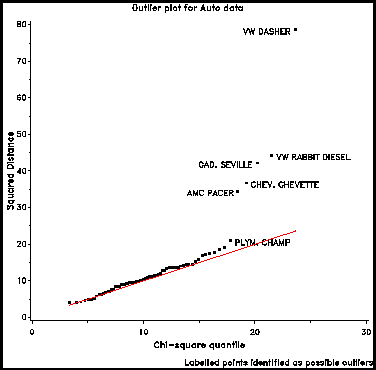Figure 8: Outlier chi-square plot for AUTO data

For further information, contact:
```   Michael Friendly
Psychology Department, York University